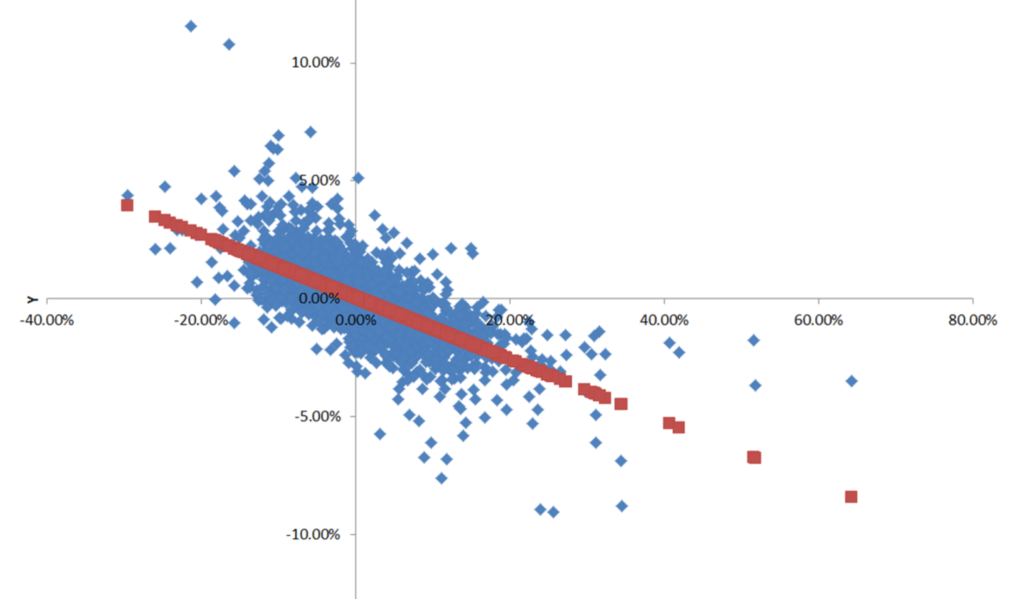# On the relationship between the S&P 500 and the CBOE Volatility Index (VIX)

Besides going over the course syllabus during the first day of class on Tuesday, January 19, we will also discuss a particularly important “real world” example of financial risk. Specifically, we will look at the relationship between stock market returns (as indicated by daily percentage changes in the SP500 stock market index) and stock market volatility (as indicated by daily percentage changes in the CBOE Volatility Index (VIX)):As indicated by this graph from page 22 of the lecture note for the first day of class, daily percentage changes on closing prices for VIX (which is the x-axis variable) and the SP500 (which is the y-axis variable) are strongly negatively correlated. The blue points represent 7,712 daily observations on these two variables, spanning the time period from January 3, 1990, through August 10, 2020. When we fit a regression line through this scatter diagram, we obtain the following equation:${R_{SP500}} = 0.0594 - 0.1126{R_{VIX}}$,

where${R_{SP500}}$ corresponds to the daily return on the SP500 index and${R_{VIX}}$ corresponds to the daily return on the VIX index. The slope of this line (-0.1156) indicates that on average, daily VIX returns during this time period were inversely related to the contemporaneous daily return on the SP500; i.e., when volatility, as measured by VIX, went down (up), then the stock market return as indicated by SP500 typically went up (down). Nearly half of the variation in the stock market return during this time period (specifically, 48.75%) can be statistically “explained” by changes in volatility, and the correlation between${R_{SP500}}$ and${R_{VIX}}$ comes out to -0.698. While a correlation of -0.698 does not imply that${R_{SP500}}$ and${R_{VIX}}$ always move in opposite directions, it does suggest that this will be the case more often than not. Indeed, closing daily returns on${R_{SP500}}$ and${R_{VIX}}$ during this period moved inversely 78.62% of the time.

You can see how the relationship between the SP500 and VIX evolves prospectively by entering http://finance.yahoo.com/quotes/^GSPC,^VIX into your web browser’s address field.